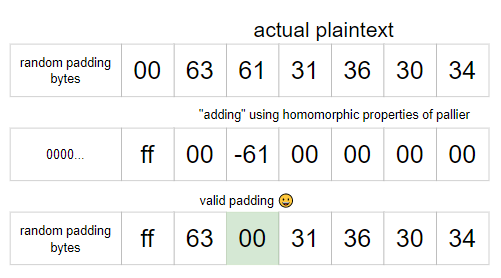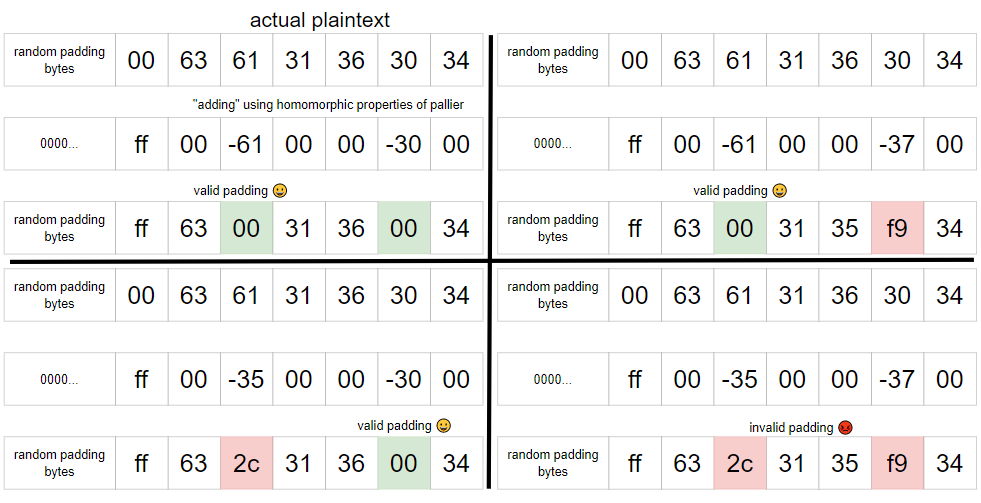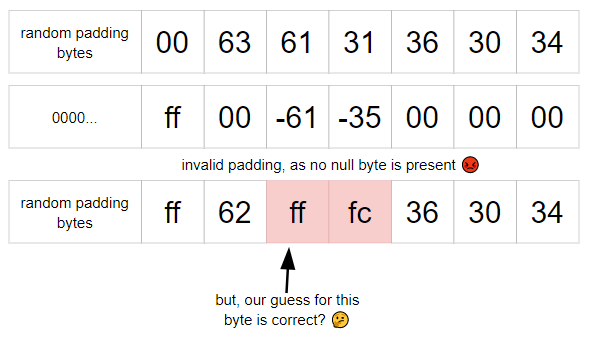# GoogleCTF 2022 - maybe someday

I played GoogleCTF 2022 with DiceGang, and we got 2nd place!This challenge created by mystiz was pretty interesting, so I wrote it up.

# maybe someday

Leave me your ciphertexts. I will talk to you later.

maybe-someday.2022.ctfcompetition.com 1337

skip code

#!/usr/bin/python3

#
# you may not use this file except in compliance with the License.
# You may obtain a copy of the License at
#
#
# Unless required by applicable law or agreed to in writing, software
# WITHOUT WARRANTIES OR CONDITIONS OF ANY KIND, either express or implied.
# See the License for the specific language governing permissions and

from Crypto.Util.number import getPrime as get_prime
import math
import random
import os
import hashlib

# Suppose gcd(p, q) = 1. Find x such that
#   1. 0 <= x < p * q, and
#   2. x = a (mod p), and
#   3. x = b (mod q).
def crt(a, b, p, q):
return (a*pow(q, -1, p)*q + b*pow(p, -1, q)*p) % (p*q)

def L(x, n):
return (x-1) // n

class Paillier:
def __init__(self):
p = get_prime(1024)
q = get_prime(1024)

n = p * q
λ = (p-1) * (q-1) // math.gcd(p-1, q-1) # lcm(p-1, q-1)
g = random.randint(0, n-1)
µ = pow(L(pow(g, λ, n**2), n), -1, n)

self.n = n
self.λ = λ
self.g = g
self.µ = µ

self.p = p
self.q = q

# https://www.rfc-editor.org/rfc/rfc3447#section-7.2.1
padding_size = 2048//8 - 3 - len(m)

raise Exception('message too long')

return b'\x00\x02' + random_padding + b'\x00' + m

if m[:2] != b'\x00\x02':
raise Exception('decryption error')

raise Exception('decryption error')

return m

def public_key(self):
return (self.n, self.g)

def secret_key(self):
return (self.λ, self.µ)

def encrypt(self, m):
g = self.g
n = self.n

m = int.from_bytes(m, 'big')

r = random.randint(0, n-1)
c = pow(g, m, n**2) * pow(r, n, n**2) % n**2

return c

def decrypt(self, c):
λ = self.λ
µ = self.µ
n = self.n

m = L(pow(c, λ, n**2), n) * µ % n
m = m.to_bytes(2048//8, 'big')

def fast_decrypt(self, c):
λ = self.λ
µ = self.µ
n = self.n
p = self.p
q = self.q

rp = pow(c, λ, p**2)
rq = pow(c, λ, q**2)
r = crt(rp, rq, p**2, q**2)
m = L(r, n) * µ % n
m = m.to_bytes(2048//8, 'big')

def challenge(p):
secret = os.urandom(2)
secret = hashlib.sha512(secret).hexdigest().encode()

c0 = p.encrypt(secret)
print(f'{c0 = }')

# # The secret has 16 bits of entropy.
# # Hence 16 oracle calls should be sufficient, isn't it?
# for _ in range(16):
#     c = int(input())
#     try:
#         p.decrypt(c)
#         print('😀')
#     except:
#         print('😡')

# I decided to make it non-interactive to make this harder.
# Good news: I'll give you 25% more oracle calls to compensate, anyways.
cs = [int(input()) for _ in range(20)]
for c in cs:
try:
p.fast_decrypt(c)
print('😀')
except:
print('😡')

guess = input().encode()

if guess != secret: raise Exception('incorrect guess!')

def main():
with open('/flag.txt', 'r') as f:

p = Paillier()
n, g = p.public_key()
print(f'{n = }')
print(f'{g = }')

try:
# Once is happenstance. Twice is coincidence...
# Sixteen times is a recovery of the pseudorandom number generator.
for _ in range(16):
challenge(p)
print('💡')
print(f'🏁 {flag}')
except:
print('👋')

if __name__ == '__main__':
main()


### challenge overview

We are given access to a server which encrypts a hashed 2 byte secret using the Paillier cryptosystem. We are asked to submit 20 ciphertexts, and then it will decrypt them all at once.

For each ciphertext, it will tell us whether it has valid padding (standard PKCS V1.5) or not.

We are then asked for the hashed secret, and if it is correct, we can continue. If we can repeat this 16 times in a row, we get the flag.

## solution

Since we are dealing with a padding oracle type challenge, we should focus on the padding checking function itself.

def unpad(self, m):
if m[:2] != b'\x00\x02':
raise Exception('decryption error')

raise Exception('decryption error')

return m


The method used to check padding is that it splits the message into two parts by splitting at the first null byte, and then checking if the length of the first part (the random part) is greater than 8 bytes. It also checks if the first two bytes are \x00\x02. Therefore, there are three ways to cause the padding to be invalid:

• corrupt the two bytes at the start of the plaintext
• place the null byte at the start of the plaintext such that the random padding is less than 8 bytes
• have no null byte in the plaintext at all, which means splitting m by a null byte will result in only one value, which will cause an error as the code expects two.

We’ll mainly focus on abusing this last method.

### paillier cryptosystem

Understanding the fine details of the Paillier cryptosystem used is not required for this challenge, although one very important thing we need to know is that Paillier has the property of homomorphic addition, that is, if we denote Paillier encryption as $P(x)$, then:

$P(a) * P(b) = P(a + b)$

We can show this by looking at Paillier in little more detail, specifically at the encryption of a message.

A keypair is generated by generating two primes $p, q$, and then computing $n = p * q$, and then a $g \in (0, n-1)$ is also chosen. The public key is the pair $(n, g)$. I won’t cover how the private key/decryption works here, since it is not very relevant for the challenge. All we need to know is that it works :)

To encrypt a message $m$, a random $r \in (0, n-1)$ is chosen, and then the ciphertext $c$ is equal to:

$c \equiv g^{m} * r^n \bmod n^2$

The $r$ value when encrypting messages does not affect what it decrypts to, it only changes the ciphertext itself.

Now, we can show the property above by multiplying the ciphertexts of two messages $m_1, m_2$. We also denote the $r$ values chosen for the encryption as $r_1, r_2$

$\begin{eqnarray} c_1 &=& g^{m_1}r^{n}\\ c_2 &=& g^{m_2}r^{n}\\ c_1c_2 &=& g^{m_1}g^{m2} * r_1^{n}r_2^{n} \bmod n^{2}\\ \end{eqnarray}$

Notice that $g^{m_1} * g^{m_2}$ turns into $g^{(m1 + m2)}$, and notice that $r_1 ^ {n} * r_2^{n}$ turns into $(r_1r_2)^{n}$. Since the $r$ values are random, a product of $r$ values will just result in another “possible” $r$ value (which we’ll denote $r_3$).

Therefore, we have:

$c_1c_2 = g^{(m1 + m2)} r_3^{n} \bmod n^{2}$

which is an encryption of $m_1 + m_2$.

As we established above, the $r$ value does not affect decryption, so it does not matter if the $r$ values are different, it will still decrypt to $m_1 + m_2$.

### leaking information

Using this homomorphic addition property, we are able to arbitrarily add values to our plaintext (which includes negative values, therefore allowing for subtraction too).

However, since we have to submit all 20 ciphertexts at once, we cannot use any sort of adaptive ciphertext attacks, where we modify the ciphertext based on the result of the query (such as binary search).

The trick to this challenge is that since we can arbitrarily subtract any value from the plaintext, we can use this to guess a value for a byte at a certain position, by subtracting a value such that it will turn into a null byte.

Since there is already a null byte in the padding, we must first add some random value such that this null byte disappears.

Then, a null byte will be present in the plaintext if our guess for the byte at that position is correct. Since the null byte is what causes the padding to be valid, the padding will only be valid if our guess is correct.Otherwise, if our guess is wrong, there will be no null byte in the plaintext, and it will error (as mentioned above).We can also extend this to testing for multiple bytes, although we will only know if at least one of our guesses is correct, and we won’t know which one that is.So, this allows us to, on each query, divide the possible plaintexts into two sets, one where the padding would be valid, and one where the padding would not be valid (the random bytes are not affected, and so do not matter). If we send 20 different queries, and find the intersection of the 20 resulting sets, we should hope to find only the correct plaintext.

Theoretically we only need 16, as there are 16 bits of entropy, however the author was very nice and gave us 4 extra, so we can use a very naive method to generate them, which is essentially just randomly generating queries that split the set of candidates in half.

### implementation details

To generate the queries, I used a greedy-type algorithm to pick the most common character (that wouldn’t cause the subset to contain above half) at a randomly chosen index, adding all candidates which had that character at the index, and repeating until the resulting subset was about half of the total number of candidates. Additionally, since the possible candidates remain the same for all rounds, we can use the same queries each time.

One thing we need to be quite careful about when generating the queries is that we cannot test consecutive bytes in the same query.

To see why this is an issue (and you might have spotted it in the diagram above), suppose we make a guess for the second byte that is too high. The subtraction will cause the first byte to decrease by one, so even if we guess the first byte correctly, the null byte will not appear, and the padding will be marked as invalid, meaning we’ll get an invalid result, even though our guess for the first byte is correct, and we would expect this to result in a successful decryption 😡.Of course, this is quite easy to fix, we just make sure that when we randomly pick an index, we ensure it isn’t adjacent to an index we have already chosen before. 1

To test the probability of success on a given round, we just need to divide the number of unique responses of the server by the total number of candidates.

Why is this the case? Remember, we are trying to guess the candidate that gave the response received by the server.

Suppose for a unique response, there are $k$ candidates out of the set of all candidates that produce the response. Then, the server has a $\frac{k}{n}$ chance of picking a candidate which produces this response, where $n$ is the number of possible candidates. We have a $\frac{1}{k}$ chance of guessing the correct candidate. Therefore, for all unique responses, there is a $\frac{k}{n} * \frac{1}{k} = \frac{1}{n}$ chance of success, regardless of $k$, and therefore the probability will just be the sum of these for all unique responses.

For a given round, the success rate is about $95.5\%$, therefore the success rate for all 16 rounds will be $95.5^{16} \approx 47.9\%$, meaning we should only need to run this one or two times to get the flag.

from pwn import *
import random
import itertools
from functools import reduce
from collections import Counter
from tqdm import tqdm

s = remote("maybe-someday.2022.ctfcompetition.com", 1337)

s.recvuntil("n = ")
n = int(s.recvline())
s.recvuntil("g = ")
g = int(s.recvline())

candidates = [hashlib.sha512(bytes(secret)).hexdigest().encode() for secret in itertools.product(range(256), repeat=2)]

def query(cts):
[s.sendline(str(x)) for x in cts]
resp = [s.recvline().decode().strip() == "😀" for _ in range(20)]
print(resp)
return resp

def generate_query():
subset = set()
indexes = set()
querying = []
unused = candidates[:]
while len(subset) < 32768: # ideally 50% in each set
index = random.randrange(1, 128) # just pick random indexes, leave the first one untouched for underflow
if all([index + k not in indexes for k in range(-1, 2)]): # check no adjacent bytes
c = Counter([cand[index] for cand in unused])
for i, (char, freq) in enumerate(c.most_common()): # greedy-type algorithm
if freq < (32768 - len(subset)):
querying.append((index, char))
[subset.add(cand) for cand in unused if cand[index] == char]
break

elif i == 15:
querying.append((index, char))
[subset.add(cand) for cand in unused if cand[index] == char]

unused = [u for u in unused if u not in subset]
fset = set(unused)
return querying, fset, subset # query itself, set when invalid padding, set when valid padding

def generate_queries():
datas = [generate_query() for _ in range(20)]
queries = [data for data in datas]
qsets = [[data, data] for data in datas]
return queries, qsets

def pallier_encrypt(m):
# raw pallier encryption without padding
r = random.randint(0, n-1)
c = pow(g, m, n**2) * pow(r, n, n**2) % n**2
return c

def calculate_ct(c, query):
querypt = (0xff << 128*8) - sum([x * 1 << (8 * (127 - i)) for i, x in query])
# first part is to get rid of the null byte part of the normal padding
# second part is our query
new_ct = (c * pallier_encrypt(querypt))
return new_ct % n**2

def check_queries(queries):
# checks how many unique responses we can get, out of the possible len(candidates)
resps = set()
for cand in tqdm(candidates):
resp = sum([2**i * (sum([cand[index] == char for index, char in query]) > 0) for i, query in enumerate(queries)])
return len(resps)/len(candidates)

find_intersection = lambda a, b : a.intersection(b)
queries, qsubsets = generate_queries() # we don't need to regenerate the queries each time
prob = check_queries(queries)
print("probability of success for one round: ", prob) # usually around 0.96
print("probability of success for all rounds: ", prob**16) # usually around 0.5

def solve_round():
s.recvuntil("=")
c = int(s.recvline())
roundqueries = [calculate_ct(c, query) for query in queries] # but we need to recalculate what we send to the server
resp = query(roundqueries)
subsets = [qsubsets[i][r] for i, r in enumerate(resp)] # selecting the subsets based on the response
secret = reduce(find_intersection, subsets) # and we find their intersection
print("intersection length: ", len(secret))
s.sendline(secret.pop()) # hopefully this is of length 1 and therefore is the secret

for roundnum in range(1, 17):
print("round ", roundnum)
solve_round()
if s.recvline().decode().strip() == "👋":
print("failed on round", roundnum)
else:
print("sice on round", roundnum)

s.interactive()


#### Flag: CTF{p4dd1n9_or4cl3_w1th_h0mom0rph1c_pr0p3r7y_c0m6in3d_in7o_a_w31rd_m47h_puzz1e}

1. If you really wanted to, you could probably find a workaround, but you’d need to take the other queries into account, and I didn’t want to implement it, so avoiding the problem entirely seemed better.